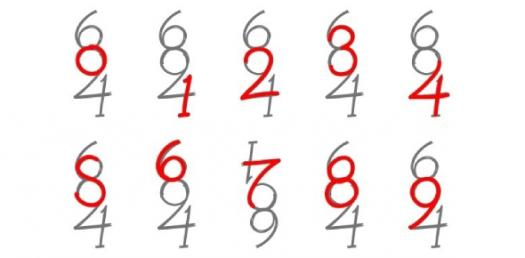# Addition Trivia Quiz: Add These Numbers And Find The Correct Answer!

48 Questions | Total Attempts: 361SettingsCreate your own QuizWelcome to this Addition Trivia Quiz, Add These Numbers And Find The Correct Answer! Math is one of the best subjects for testing how sharp a person is since it has rules that are easy to follow and allowing for a level field to test. If you are feeling up to the task, do press the start button and test yourself.

• 1.
9+4=
• 2.
5+6=
• 3.
2+9=
• 4.
6+5=
• 5.
6+7=
• 6.
5+5=
• 7.
4+2=
• 8.
1+9=
• 9.
4+7=
• 10.
5+8=
• 11.
7+6=
• 12.
5+8=
• 13.
3+6=
• 14.
7+3=
• 15.
4+4=
• 16.
2+7=
• 17.
7+6=
• 18.
4+8=
• 19.
1+9=
• 20.
8+5=
• 21.
9+4=
• 22.
3+9=
• 23.
2+9=
• 24.
6+6=
• 25.
7+5=
Related TopicsBack to top
×

Wait!
Here's an interesting quiz for you.